# Mean-square approximation of a function

(diff) ← Older revision | Latest revision (diff) | Newer revision → (diff)

An approximation of a functionby a function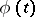, where the error measureis defined by the formula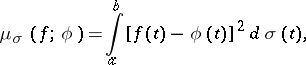where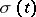is a non-decreasing function ondifferent from a constant.

Let(*)

be an orthonormal system of functions onrelative to the distribution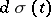. In the case of a mean-square approximation of the functionby linear combinations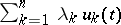, the minimal error for everyis given by the sums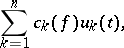where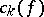are the Fourier coefficients of the function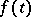with respect to the system (*); hence, the best method of approximation is linear.

How to Cite This Entry:
Mean-square approximation of a function. Encyclopedia of Mathematics. URL: http://encyclopediaofmath.org/index.php?title=Mean-square_approximation_of_a_function&oldid=17600
This article was adapted from an original article by N.P. KorneichukV.P. Motornyi (originator), which appeared in Encyclopedia of Mathematics - ISBN 1402006098. See original article